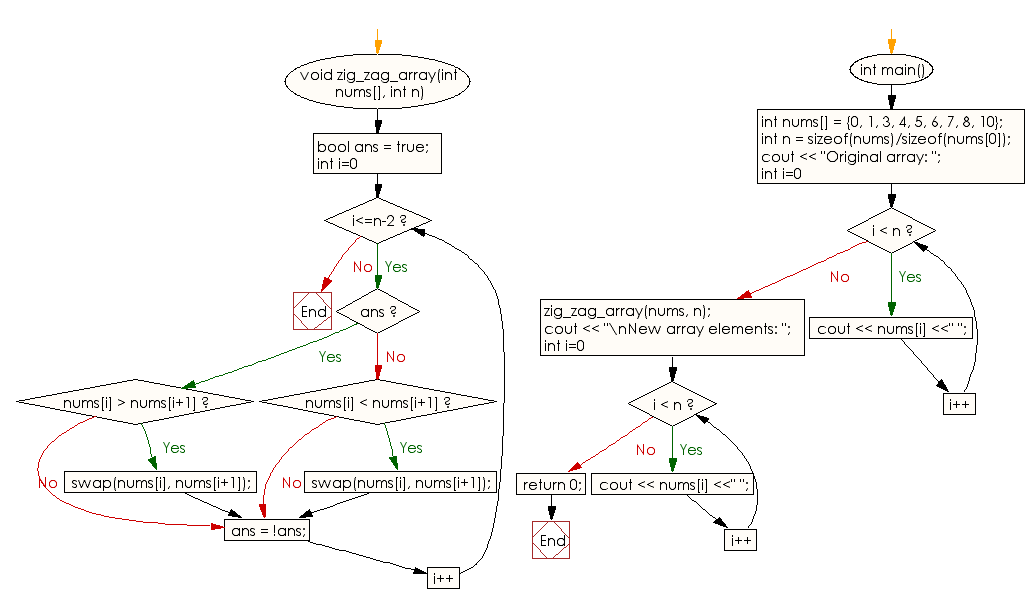﻿ C++ : Rearrange the values of an array in zigzag way# C++ Exercises: Rearrange the elements of a given array of integers in zig-zag fashion way

## C++ Array: Exercise-12 with Solution

Write a C++ program to rearrange the elements of a given array of integers in zig-zag fashion way.
Note: The format zig-zag array in form a < b > c < d > e < f.

Sample Solution:

C++ Code :

``````#include<iostream>
using namespace std;

void zig_zag_array(int nums[], int n)
{
bool ans = true;

for (int i=0; i<=n-2; i++)
{
if (ans)
{
if (nums[i] > nums[i+1])
swap(nums[i], nums[i+1]);
}
else
{
if (nums[i] < nums[i+1])
swap(nums[i], nums[i+1]);
}
ans = !ans;
}
}

int main()
{
int nums[] = {0, 1, 3, 4, 5, 6, 7, 8, 10};
int n = sizeof(nums)/sizeof(nums);
cout << "Original array: ";
for (int i=0; i < n; i++)
cout << nums[i] <<" ";
zig_zag_array(nums, n);
cout << "\nNew array elements: ";
for (int i=0; i < n; i++)
cout << nums[i] <<" ";
return 0;
}
``````

Sample Output:

```Original array: 0 1 3 4 5 6 7 8 10
New array elements: 0 3 1 5 4 7 6 10 8
```

Flowchart:C++ Code Editor:

What is the difficulty level of this exercise?

Test your Programming skills with w3resource's quiz.

﻿